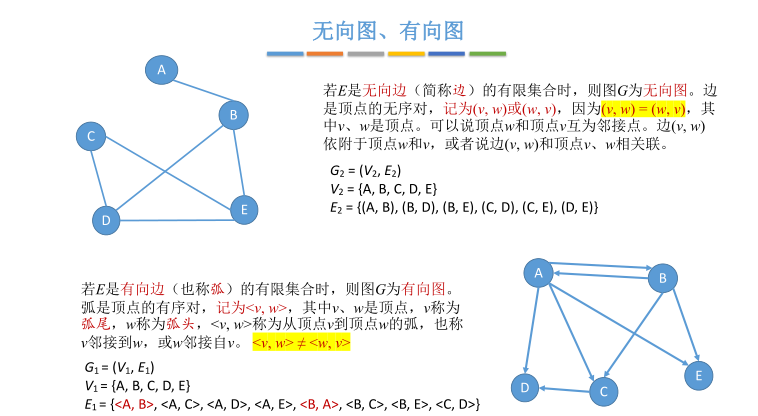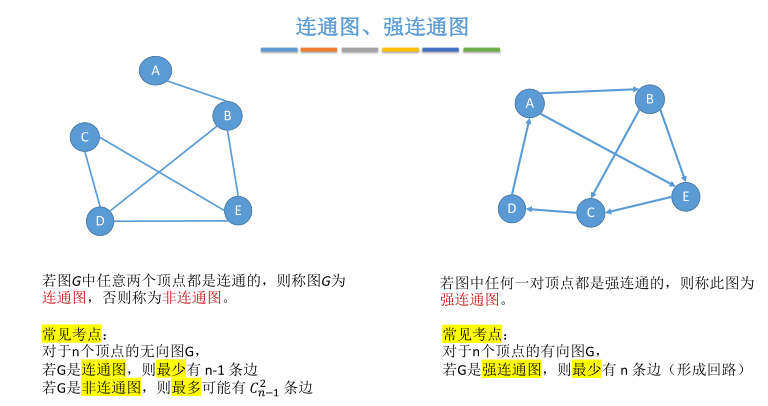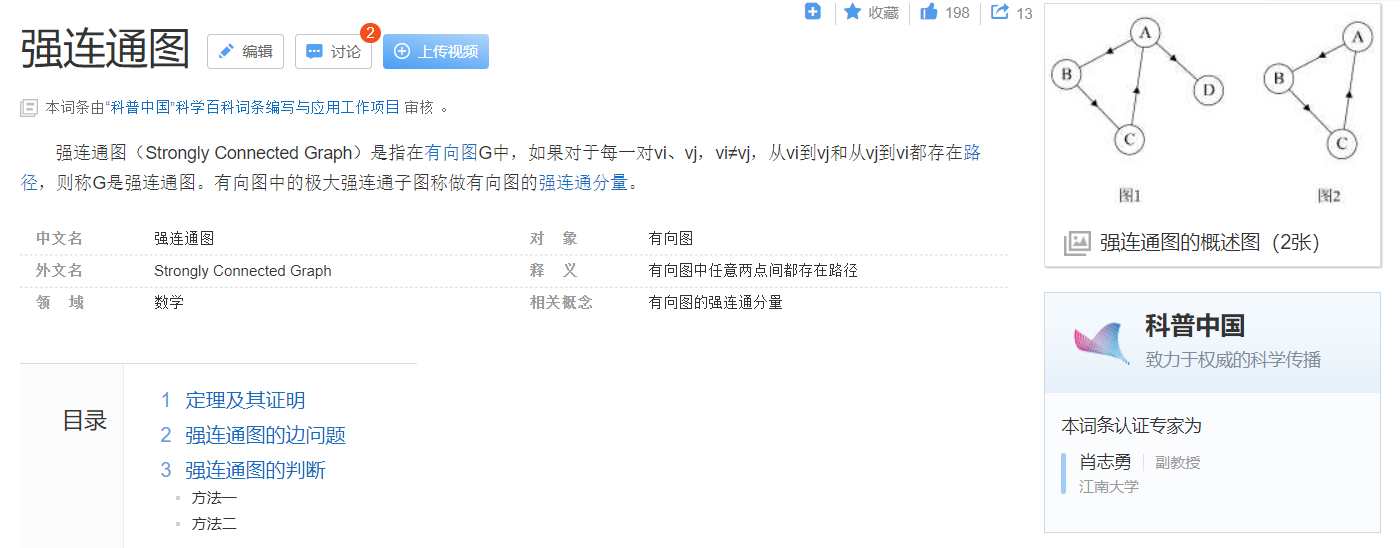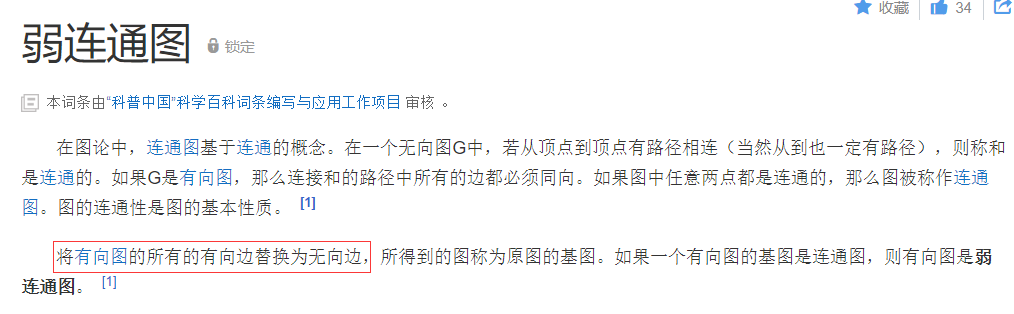展开全部

下面是这强连通、单向连通、弱连通、不连通的定义：

强连通图：有向图 G=(V,E) 中，若对于V中任意两个不同的顶点 x和 y，都存在从x到 y以及从 y到 x的路径，则称 G是强连通图。相应地有强连通分量的概念。强连通图只有一个强连通分量，即是其自身；非强连通的有向图有多个强连分量。

单向连通图：设G=是有向图，如果u->v意味着图G至多包含一条从u到v的简单路径，则图G为单连通图。

弱连通图：将有向图的所有的有向边替换为无向边，所得到的图称为原图的基图。如果一个有向图的基图是连通图，则有向图是弱连通图。

初级通路：通路中所有的顶点互不相同。初级通路必为简单通路，但反之不真。

在图论中，连通图基于连通的概念。在一个无向图 G 中，若从顶点i到顶点j有路径相连(当然从j到i也一定有路径)，则称i和j是连通的。如果 G 是有向图，那么连接i和j的路径中所有的边都必须同向。

如果图中任意两点都是连通的，那么图被称作连通图。如果此图是有向图，则称为强连通图(注意：需要双向都有路径)。图的连通性是图的基本性质。扩展资料：

强连通图的边问题：

有n个顶点的强连通图最多有n(n-1)条边，最少有n条边。

1、最多的情况：即n个顶点中两两相连，若不计方向，n个点两两相连有n(n-1)/2条边，而由于强连通图是有向图，故每条边有两个方向，n(n-1)/2×2=n(n-1)，故有n个顶点的强连通图最多有n(n-1)条边。

2、最少的情况：即n个顶点围成一个圈，且圈上各边方向一致，即均为顺时针或者逆时针，此时有n条边。

求无向图的连通分量：

作为遍历图的应用举例，下面我们来讨论如何求图的连通分量。无向图中的极大连通子图称为连通分量。求图的连通分量的目的，是为了确定从图中的一个顶点是否能到达图中的另一个顶点，也就是说，图中任意两个顶点之间是否有路径可达。

对于连通图，从图中任一顶点出发遍历图，可以访问到图的所有顶点，即连通图中任意两顶点间都是有路径可达的。

展开全文弱连通和强连通
• 前言网上现存$$60\%$$的文章都有明显的误区，本文章经过多次修改，能保证正确性本文涉及连通分量、弱连通分量、割点、割边、边双、点双，属于基本图论范畴在有着直接关联的基础上又有所不同，本文基于把抽象的数组...

前言

网上现存$$60\%$$的文章都有明显的误区，本文章经过多次修改，能保证正确性

本文涉及强连通分量、弱连通分量、割点、割边、边双、点双，属于基本图论范畴

在有着直接关联的基础上又有所不同，本文基于把抽象的数组转换为在图上的意义，旨在让初学者能更轻松地理解并区分差别

为避免各个板子的差别过大，在正确的基础上尽量保证代码的相似性

如果您之前学过，可能与您的定义有所不同，故请在看完每个算法下面的代码后再进行文字阅读

文字中某个词语后出现带圆框的数字，如①②，这些词语将会在文字下方有详细的注释，方便阅读

前置

我们简略地定义$$dfs$$树为遍历路程中路径所组成的一棵树，注意下面说的儿子、叶子节点、子树边界$$($$与子树直接相连的外部节点$$)$$等用法都从此基础上得出

如下图及$$dfs$$树，$$3,7$$为$$1$$的儿子，叶子节点为$$2,5,6,8$$，$$7$$的子树边界为$$\{1\}(1$$与$$7$$和$$8$$直接相连$$)$$，如果新加一条边$$(3,4)$$，则$$7$$的子树边界为$$\{1,3\}$$有向图

强连通分量

定义：有向图中某个点集中的点互相能到达的分量为强连通分量

为方便理解我们采取归纳法：找到完整强连通分量后立即染色

定义$$dfn_u$$：表示$$dfs$$中$$u$$的时间戳；初始化为第几个被遍历到的点。

定义$$low_u$$：表示$$u$$能到达且在$$u$$子树边界的未染色的最小时间戳①$$($$设代表该最小时间戳点的点为$$x$$，可证明$$x$$一定能与$$u$$组成强连通分量②$$)$$；初始化为$$dfn_u$$。

①：显然代表该最小时间戳的不为$$u$$的子树$$($$除$$u)$$，因为子树内的时间戳$$u$$已经为最小的了。故$$u$$的子树并不影响$$low_u$$，真正影响的是$$u$$的子树外，与$$u$$子树有接触，且未染色的。

②：$$($$下图为例$$)x$$位于$$f$$的左子树，$$x$$所在完整强连通内所有节点不止在左子树$$($$否则就染色了$$)$$，$$x$$至少能与$$f$$组成强连通分量。故$$x$$一定能与$$u$$组成强连通分量：$$f\rightarrow u\rightarrow x\rightarrow f$$。具体做法：在$$u$$的子树遍历完后，$$low_u=dfn_u$$则把栈顶到该点的区间染色$$($$与子树外单向联通，那$$u$$的子树未处理部分与$$u$$组成强连通分量$$)$$，否则要等回到某个祖先后染色才能分量的完整

code

也可更换第$$7$$行代码为：

else if(visit[v]) low[u]=std::min(low[u],low[v]);

//此时定义low：能与u组成强连通分量(未染色)的最小时间戳

void Tarjan(LL u){

dfn[u]=low[u]=++tim; sta[++top]=u; visit[u]=true;

LL v(dis[i].to);

if(!dfn[v]){

Tarjan(v); low[u]=std::min(low[u],low[v]);

}else if(visit[v]) low[u]=std::min(low[u],dfn[v]);

}

if(low[u]==dfn[u]){

LL now; ++nod;

do{

now=sta[top--]; col[now]=nod; visit[now]=false;③

}while(now!=u);

}

}

③：$$visit$$清空：强连通分量是建立在有向图上的，$$u\rightarrow v,v\nrightarrow u$$时，如果之前先遍历过$$v$$，则已经把$$visit$$清空了，此时$$u$$不受$$v$$的任何影响

弱连通分量

定义：同一弱连通分量里的任意两个点$$x,y$$，保证至少一方能到达另一方

想象一下某个弱连通分量进行强连通缩点后的样子？能两两到达的肯定存在于同一个大点中了，剩下的肯定是单向联通，故一定是一条单直链

性质：某一点可能属于多个弱连通分量，显然，属于强连通分量的两点一定属于同一弱连通分量

做法：在强连通缩点后的$$DAG$$图中，每一条链就是一个弱连通分量

无向图

为了割点与桥的统一计算，在无向图中我们不管父亲$$($$类似树的遍历，遇到$$f$$则$$continue)$$，稍后会说明原因

且重新定义：

定义$$low_u$$：$$u$$的子树与子树外接触的最小时间戳$$($$除$$(u,f)$$的影响,因为在遍历$$u$$时遇到$$f$$会跳过$$)$$

如下图，蓝边为$$dfs$$树，标号为时间戳，$$6$$的子树为$$\{6,7,8,9\}$$，子树与子树外的接触为$$(6,3),(7,5),(9,2)$$，故$$low_6=2$$割点

定义：无向图中，将该点从原图中拿掉后，连通分量数量增加

想象一下割点在图上的样子：一个点至少夹在两个互不接触 (( 不考虑该点的连接作用 )) 联通块之间为了便于理解，先想一下暴力做法④：特判每一个点，如果该点至少有两个儿子则说明为割点$$($$这些儿子所属的子树互不接触，否则仅需遍历一次，而该点位于子树之间，显然割掉后连通块个数=儿子个数$$)$$。时间复杂度$$O(nm)$$

④：因为判断儿子得把整个子树全跑一遍。而每一次判断的儿子个数仅对根有效。因为根肯定得把一个子树的点遍历完才能回溯；其他的点由于顺序关系，入度也会成为一个儿子$$($$对于无根树$$)$$，实际上入度上方可能与儿子有接触，故不能一次性判断。比如下面的这幅图，从$$1$$开始遍历则$$3$$有两个儿子；但从$$3(root)$$开始遍历：$$3\longrightarrow 2\longrightarrow 1\longrightarrow 12\longrightarrow 13\longrightarrow9\longrightarrow8\longrightarrow6\longrightarrow7\longrightarrow5\longrightarrow4\longrightarrow10\longrightarrow11$$，最后得到的是$$3$$只有一个儿子，所以$$3$$不为割点转换成条件：对于$$(u,v),fa_v=u$$，在割掉$$u$$后，$$v$$的子树与外界无任何接触。也就是$$v$$的子树仅与外界的$$u$$接触，则当割掉$$u$$后，多生成了一个联通分量。

抽象成代码：$$u\in Articulation Point \longrightarrow low[v]>=dfn[u]$$

细节：如果我们首先遍历$$u(root)$$，则无论怎样$$low_v$$都会$$≥dfn_u(dfn_u=1)$$，则根据定义是把$$u$$判断为割点，其实不然⑤。

⑤：有时我们发现根不会为割点，这是为什么呢?因为$$u$$的子树就是所有点，故没有外界，也就是说特判一定满足，故该特判对其无效。

所以需要判断的是$$u$$是否有至少两个儿子$$($$原理就是上面的暴力做法$$)$$，否则就为无根树上的叶子节点了$$($$也就是边界$$)$$

code

void Tarjan(LL u,LL mr,LL f){

LL rc(0);

dfn[u]=low[u]=++cnt;

LL v(dis[i].to);

if(v==f) continue;

if(!dfn[v]){

Tarjan(v,mr,u);

low[u]=min(low[u],low[v]);

if(low[v]>=dfn[u]&&u!=mr)

cut[u]=true;

if(u==mr)

rc++;

}else low[u]=min(low[u],dfn[v]);

}

if(u==mr&&rc>=2)

cut[mr]=true;

}

定义：又称为割边，将该边从原图中拿掉后，连通分量数量增加

想象一下桥在图上的样子：一条边被两个不接触$$($$不考虑这条边的连接作用$$)$$的联通块夹在中间。

如下方，两个"$$+$$点"间的为桥为了便于理解，先想一下暴力做法：枚举每一条边$$(x,y)$$从$$x$$节点出发不经过该边遍历一次，如果不能到达$$y$$则该边为桥。时间复杂度$$O(m^2)$$

转换成条件：对于桥$$(u,f),fa_u=f$$的，割掉$$(u,f)$$后，$$u$$的子树外界无任何接触。也就是除$$(u,f)$$外，$$u$$的子树的边仅局限在内部，相当于u的子树被一根线挂在$$f$$节点上。

抽象成代码：$$(u,v)\in Bridge\longrightarrow low[v]>dfn[u]$$

void Tarjan(LL u,LL f){

dfn[u]=low[u]=++cnt;

LL v(dis[i].to);

if(v==f) continue;

if(!dfn[v]){

Tarjan(v,u);

low[u]=min(low[u],low[v]);

if(low[v]>dfn[u]){

e[++tot]=u; e[tot]=v;

}

}else low[u]=min(low[u],dfn[v]);

}

}

其实割点是可以考虑父亲的影响，而桥绝对不能

因为$$f$$为割点仅需要考虑$$u$$的子树最多遍历到$$f$$，也就是说$$(u,f)$$这条边对判断起不到任何影响

而桥$$(f,u)$$需要：除开这条边，$$u$$的子树最多遍历到$$u$$。如果考虑父亲的影响，$$low_u$$一定会受$$dfn_f$$的影响$$(low_u=dfn_f)$$，特判起来会变得十分麻烦

故为了代码的方便，在割点割边时不考虑父亲

边双连通分量

定义：简写为边双，同一边双内，点与点的边集中无桥

如下图，每种颜色的点为一个边双，之间由桥隔开具体做法

两次遍历：这个就比较简单了，直接找出所有的桥删掉，然后遍历一遍染色就行了，因为桥已经被全部删掉，故每种颜色的分量的边集中肯定无桥

一次遍历：桥的定义入手，考虑桥$$(f,u)$$，$$u$$的子树局限于内部，故满足$$low_u=dfn_u$$；而同属$$u$$的边双内的任意点$$x$$，由于无桥，肯定不会局限于$$x$$的子树，故满足$$low_x≠dfn_x$$。与强连通分量的做法类似，判断$$dfn_u=low_u$$，把压进栈里的点取出来染色即可

void Tarjan(LL u,LL fa){

dfn[u]=low[u]=++tim; sta[++top]=u;

LL v(dis[i].to);

if(v==fa) continue;

if(!dfn[v]){

Tarjan(v,u); low[u]=std::min(low[u],low[v]);

}else low[u]=std::min(low[u],dfn[v]);

}

if(low[u]==dfn[u]){

LL now; ++nod;

do{

now=sta[top--]; col[now]=nod;

}while(now!=u);

}

}

点双连通分量

定义：简写为点双，对于同属一个点双的任意点，删除后，该分量中的点仍能互相到达；或者说仅对于该分量而言，无割点。具体做法：

依旧从割点的定义入手：割点将原图分成互不相连的多个联通块，显然每个联通块本身已经是一个点双了，但不完整，因为相邻的割点在边界，如果与联通块共同组成一个新联通块，割掉后也不会另外产生联通块$$($$相当于该连通块上的叶子节点$$)$$，所以需要加上来才完整$$($$故割点是会同时存在于多个点双中的$$)$$

我们怎么染色呢？可以发现在$$dfs$$树中，割点$$u$$在进行与儿子节点的染色时会分给多个点双，而在遍历完儿子后，与祖先将仅产生一个完整点双⑥。所以当$$u$$为割点，给儿子节点染色时，取到儿子借点就够了，栈中保留$$u$$，等到$$u$$的某个祖先后再取出来。

新建一个节点来维护某个点双，该点向该点双的每个点连一条边$$($$当然这是建立在新图上的$$)$$，这就是广义圆方树

⑥：如下图void Tarjan(LL u){

dfn[u]=low[u]=++tim; sta[++sta]=u;

LL v(G1.dis[i].to);

if(!dfn[v]){

Tarjan(v);

low[u]=min(low[u],low[v]);

if(low[v]>=dfn[u]){

do{

now=sta[sta--];

}while(now!=v);

}

}else low[u]=min(low[u],dfn[v]);

}

}

我们把每个点双看作一个分量

分量无割点：说明整个联通块就是一个点双，建两个出口，随便割一个由于点双的性质所有点都能出去

分量有一个割点：在除割点的地方建一个出口，割掉割点直接去分量里的出口，割掉出口通过割点跑到其他分量的出口中

再具体点就相当于多个点双构成了一棵树$$($$仅一个节点的树除外$$)$$，而我们仅在叶子节点建出口

显然仅割点会对除本身以外的访问有影响，影响为多个分支所跨该割点访问数，

记分支的节点数分别为$$size_1,size_2,...,size_k$$，$$sum=\sum\limits_{i=1}^k size_i,ans=\sum\limits_{i=1}^k size_i\cdot(sum-size_i)+(n-1)*2$$

其实就是求：多个点双构成的树，$$x,y$$所在节点间的割点

首先得所属节点不同：$$low_y>=dfn_x$$

其次得保证$$u$$是位于期间的割点：$$dfn_u<=low_v$$

且$$u$$的该分支$$v$$，$$y$$存在于子树$$v$$内：$$dfn_v<=dfn_y$$

纯奇环偶环通过dfs树上，染色判断(由于偶环可能有两个奇环，通过一点相交，dfs树上并不能判完)

两环如果相交必定形成偶环，由于不可以重复经过边，把每个边双提出来判断一下是否存在两个环以上即可

展开全文弱连通和强连通
• 连通图、强连通图、弱连通图

千次阅读 多人点赞 2021-03-12 09:28:40
考研复试复习到离散数学的时候一道选择题判断给出的图是连通图还是强弱连通图，虽然在数据结构中学习过这方面的知识，不过当时感觉知识点太小，就没有太注意，所以今天回看王道的数据结构的资料，...3、弱连通图 ...

考研复试复习到离散数学的时候一道选择题判断给出的图是连通图还是强弱连通图，虽然在数据结构中学习过这方面的知识，不过当时感觉知识点太小，就没有太注意，所以今天回看王道的数据结构的资料，进行如下总结，一下资料来自王道。
1、有向图和无向图（很好判断）2、强连通图这里我们直接看百度百科怎么解释的强连通图3、弱连通图展开全文• 判断一个图是否为连通图、单向连通图、弱连通图。输入为有向图的邻接矩阵。输入输入有若干行第一行为正整数N(0接下来N行，每行有N个数据，每个数据以空格分隔，代表邻接矩阵。注意：输入的都是连通图。输出输出有...

判断一个图是否为强连通图、单向连通图、弱连通图。输入为有向图的邻接矩阵。

输入

输入有若干行

第一行为正整数N(0

接下来N行，每行有N个数据，每个数据以空格分隔，代表邻接矩阵。

注意：输入的都是连通图。

输出

输出有一行，数字1,2,3

1代表强连通图

2代表单向连通图

3代表弱连通图

测试输入

3

1 1 1

1 1 1

1 1 1

测试输出

1

源代码

#include

#define N 305

int main()

{

int a[N][N];

int i,j,k,n;

scanf("%d\n",&n);

for(i=0;i

for(j=0;j

scanf("%d",&a[i][j]);

for(i=0;i

for(k=0;k

for(j=0;j

if((a[i][k]!=0)&&(a[k][j]!=0))

a[i][j]=1;}

for(i=0;i

for(j=0;j

{

if((a[i][j]==0)&&(a[j][i]==0)){

printf("3\n");return 0;}

if(a[i][j]+a[j][i]==1){

printf("2\n");return 0;}

}

printf("1\n");

}

展开全文弱连通和强连通
• 连通和弱连通的概念只在有向图中存在。 连通图： 在有向图中, 若对于每一对顶点v1和v2, 都存在一条从v1到v2和从v2到v1的路径,则称此图是连通图。 弱连通图： 将有向图的所有的有向边替换为无向边，所得到的图...
•图 连通性
• 的结果判断其输入输出构成的有向图的强连通性（不需画出有向图，只需判断强连通性，）。 输入输出构成的有向图有2N个顶点，如果有b=f(a)表明从顶点a到顶点b有有向路径。 强连通（Strongly Connected）是指一个有向...
• "弱连通\n" ) ; return 1 ; } int main ( ) { int n ; printf ( "请输入结点个数:" ) ; scanf ( "%d" , & n ) ; int AdjMarix [ MAX ] [ MAX ] ; printf ( "请输入邻接矩阵:\n" ) ; for ( ...算法 c语言
• 对于可达矩阵P来说，如果P的所有元素均为1， 则所给的有向图是强连通的；对于P的所有元素（除主对角线元素外）Pij来说， 均有：Pij+Pji>0，则所给有向图是单向连通的。当所给有向图既不是强连通的，又不是单向...
• 连通、弱连通

千次阅读 2015-04-06 18:09:16
有向图强连通分量在有向图G中，如果两个顶点vi,vj间（vi>vj）有一条从vi到vj的有向路径，同时还有一条从vj到vi的有向路径，则称两个顶点强连通(strongly connected)。如果有向图G的每两个顶点都强连通，称G是一个...
• 【离散数学】单向连通和弱连通的区别

万次阅读 多人点赞 2016-07-21 19:51:32
单向连通一定是弱连通的。but，弱连通不一定是单向连通~~ 举个栗子~   是弱连通~但不是单向连通~因为a和b结点，a不能到b，b不能到a~~ 概念：单向连通图 如果有向图中，对于任意节点v1和v2，至少存在从v1到v2...读书笔记
• 连通图：在无向图中，从任意一个结点出发都能到达任意一个结点，那么称该无向图为连通图。 强连通图：在有向图中，从任意一个结点出发...极大连通子图：如果无向图的连通子图包含它的原图中所有它自身有关的边，那么数据结构
• 强连通分量是有向图相关 关于连通分量 连通分量的定义 无向图中的一个点集，点集中的任意一对点都可以互相到达，且点集外的点点集中任意一点都不能互相到达。 举个栗例子 这个无向图中存在一个连通分量（显然任意...
• 弱连通图：如果不是连通图，将有向图变成无向图，如果无向图是连通，那么有向图是弱连通。 三、代码如下 // graphics_judge.cpp : 此文件包含 "main" 函数。程序执行将在此处开始并结束。 // #include...
• 强连通分量

2016-11-21 14:08:53
连通分量@(算法学习)首先明白，这个概念是在有向图中的，其次是，如何有效寻找。...至于相对的弱连通，则抹去方向，相连即可。那么，道理很简单，如何应用呢？答案是先找回路，然后在回路的基础上，扩展结点。因为强连通分量
• 有向图 概念 相较无向图，有向图的边具有方向性 头，尾 重边的概念到有向图中变成了平行边，要注意这里是要同头同尾的 有向图中，没有自环和平行边，则称图为简单有向图 ...强连通(双向连通) u可达v，v可达u .有向图 强连通算法 强连通
• 强连通分量（超详细！！！）

千次阅读 多人点赞 2019-09-23 07:16:19
一、定义 ...有向非强连通图的极大强连通子图，称为强连通分量。 图中，子图{1,2,3,4}为一个强连通分量，因为顶点1,2,3,4两两可达。{5},{6}也分别是两个强连通分量。 二、tarjan算法时间复杂度是O...
• 有向图的强连通分量。 例题 题目链接：http://poj.org/problem?id=2186 vjudge：https://vjudge.net/problem/POJ-2186 题目大意： 有一群牛，总数为N(N<=10000)。 题目数据为牛之间的关系，比如说1仰慕2，2仰慕3...算法 C++ 强连通
• 最近看了看类的相关题，感觉简单的题很简单，但是难题的转化如果对概念不清楚，很难写，我怕我...（1） 有向图的强联通分量 （2） 无向图的双联通分量（求割点，桥） （3） 最近公共祖先 这里前两类问题比较相似，而...acm 数据结构 算法
• 文章目录无向图割点、桥、双连通分量Tarjan算法求割点和桥（割边）代码：边双连通分量 和 点双连通分量代码边双连通分量 和 点双连通分量 的缩点有向图的弱连通与强连通强连通分量Kosaraju算法Tarjan算法代码： ...割点 强连通
• 利用Lyapunov稳定性理论研究了自主群体动态行为的稳定性,证明了在弱连通网络拓扑结构中,当系统中所有的自主体都不能获得领航者信息时,所有的自主体能够取得群集运动;假设系统中至少有一个自主体可获得领航者信息时,...
• 由于上一道题涉及了环，所以我当时就在纠结一个问题，强连通图是否一定可以是环形的？（就是说强连通图是否一定是欧拉图？= 是否一定有欧拉回路？= 是否一定有一笔画的环形路线？） 现在，我给出答案，不一定。下面...
• 但是对于有向图，DFS不能直接求最大连通子图，因为两个节点之间并不是双向联通的，从a->b,不一定可以从b->a，这个时候我们介绍一种新的算法，tarjan tarjan() 首先我们考虑为什么一个图会强连通，这是因为图中...
• 本文从多个角度去讨论了强连通分量算法的设计思路，并重点分析了算法中的图转置操作的意义。 本文的内容是基于算法导论22.5节的深度分析，编写日期2019/1/22,20日掌握算法导论day13   【为什么要转置图G？】 ...
• 这一周，我们开始研究社区发现（Community Detection）算法，并了解强连通分量（Strongly Connected Components，SCC）算法，该算法根据关系的方向定位节点组，其中每个节点都可以从同一组中的每个其他节点到达。...
• 题目：有向图的邻接表存储强连通判断先把邻接表转换成邻接矩阵/xyx如果有向图强联通，则其必能构成环，即g[i][i+1]必不为0，g[n-1]也不为0(其实是错的，但是oj是这样认为的。。。)#include&lt;iostream&...
• 强连通分量是针对有向图定义的，为了区别无向图的联通分量的概念。在一个强连通分量中，任意两点都相互可达。 这个图中就存在三个强连通分量，分别{0}、{1}、{2}，因为这三个点相互不可达，但是自己到自己还是可到达......